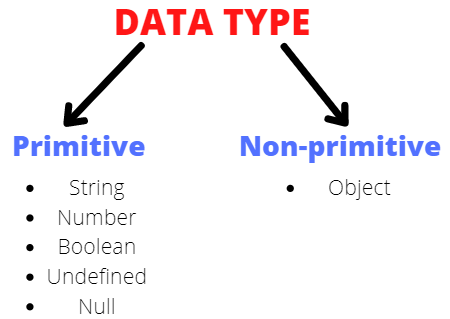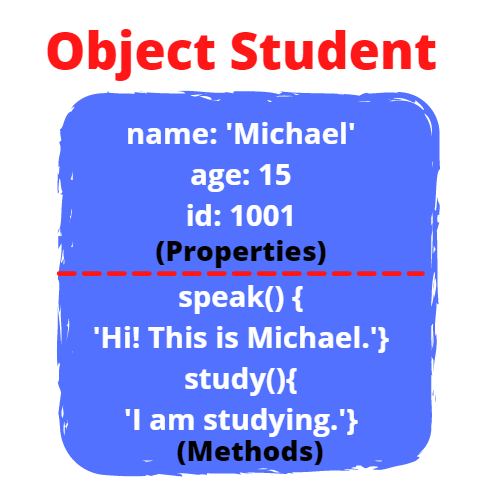# Objects in JS

## Introduction

Objects in JS is a critical concept and is widely used. You should be very clear with it before you move on to learn more advanced concepts in JavaScript.

So what is an Object? The object is a standalone non-primitive data type in JavaScript. It is used to store unordered collections of data or properties. Each of these properties is basically key and value pairs. In this blog, we will learn how to create objects, access objects, remove certain properties, and a lot more.

Let us now get started with Object in JS:

## Data Types

There are two types of data types in JavaScript:

Primitive and Non-primitive:

Primitive and Non-primitive data types are two different ways to store data in memory in JS. The main difference between the two data types is that - primitive data types are stored by value while the latter is stored by reference.

There are five types of primitive data types - stringnumber, booleanundefined, and null. Whereas object falls under non-primitive type.An object falls under the Non-primitive category.

Objects best represent real-life entities. For understanding objects better, let us take an example, imagine a student. Now what properties or features can a student have? They can have a name, age, and student id. What actions can the student do? They can speak, study and a lot more.

In JavaScript, the exact representation of a student object will look somewhat like this:## Definition

An object is an entity with a set of properties(primitive values and objects) and behaviors(methods).

The properties have a key-value pair relationship, where the keys correspond to a specific value. It is similar to an unordered map in C++.

## Creating Object in JS

To create an object in JavaScript, we can use one of the following ways:

1. Using an object literal
2. Using the keyword ‘new’
3. Using constructor

Let us have a look at them one by one:

### Object literal

Object literal refers to a comma-separated list of key-value pairs. Using it we can define and create objects at the same time.

In this example we created a Student object with name,age and id properties.

``````let Student = {
name: 'Michael',
age: 15,
id: 1001
}``````

### ‘new’ keyword

Here a new object student is created using a new keyword and then we are adding name and age property to the student.

``````const student = new Object();
student.name = 'Michael';
student.age = 15;``````

### Constructor

``````function Student(name, age, id) {
this.name = name;
this.age = age;
this.id = id;
}
const student1 = new Student('John', 14, 1201);
const student2 = new Student('Michael', 15, 1001);
console.log(student1);
console.log(student2);``````
• The function Student(){} is the object constructor function. We can create objects of the same type by calling that function along with a new keyword.
• Here student1 and student2 are two Student objects, with different names, ages, and ids.

Output:

``````Student { name: 'John', age: 14, id: 1201 }
Student { name: 'Michael', age: 15, id: 1001 }``````

Let us dig a little deeper and see how to access the object we just created.

Here we are creating a Student object and viewing the entire object by using console.log.

``````let Student = {
name: 'Michael',
age: 15,
id: 1001,
}
console.log(Student);``````

Output:

``{ name: 'Michael', age: 15, id: 1001 }``

NOTE:

We can also add properties for our object outside the curly brackets,

``````let Student = {
name: 'Michael',
age: 15,
id: 1001,
}
Student.email = 'abc@xyz.com';
console.log(Student);``````

Output:

``{ name: 'Michael', age: 15, id: 1001, email: 'abc@xyz.com' }``

But what if we want to see only the name of the student and not the other details?

To access  a specific property of an object, we can use,

1. dot(.) operator
2. square([ ]) brackets

### Accessing through Dot Operator

``````let Student = {
name: 'Michael',
age: 15,
id: 1001,
}
console.log(Student.name);``````

Output:

``Michael``

### Accessing through Square brackets

``````let Student = {
name: 'Michael',
age: 15,
id: 1001,
}
console.log(Student['name']);``````

Output:

``Michael``

But which method should you use to access specific properties of objects?

The simple answer is, you can use the dot operator as it is much more convenient than using square brackets. But there are some instances in which you have no choice other than to use square brackets. In these particular cases, using the dot operator will cause your code to throw an error.

If the name of the key is a number and not a string:

``````let Student = {
name: 'Michael',
age: 15,
id: 1001,
20: 'twenty',
}
console.log(Student['20']);``````

Output:

``twenty``

Now if we try to do the same thing utilising the dot operator, the program will throw an error.

``console.log(Student.20);``

Output:

``````console.log(Student.20);
^^^^^^^

SyntaxError: missing ) after argument list
console.log(Student.20);

^^^^^^^``````

If the name of the key has space-separated strings:

``````let Student = {
name: 'Michael',
age: 15,
id: 1001,
20: 'twenty',
'full name': 'Michael Doe'
}
console.log(Student['full name']);``````

Output:

``Michael Doe``

NOTE: It is not a good practice to use variable names which have spaces.

When the key name comes from a variable:

``````let Student = {
name: 'Michael',
age: 15,
id: 1001,
20: 'twenty',
'full name': 'Michael Doe'
}
var x = 'name';
console.log(Student[x]);``````

Output:

``Michael``

If you try to use the dot operator, the output comes out to be undefined

``console.log(student.x);``

Output:

``undefined``

NOTE: If you try to access a property which is not defined inside the object, the output shows ‘undefined’.

``````let student = {
name: 'Michael',
age: 15,
id: 1001,
}
console.log(student.gender);``````

Output:

``undefined``

## Existence of a property

To check if a property exists inside an object or not, in operator is used.

Syntax: property in object

If the property exists the operator returns true, otherwise false.

``````let Student = {
name: 'Michael',
age: 15,
id: 1001,
20: 'twenty',
'full name': 'Michael Doe'
}
console.log('gender' in Student);
console.log('age' in Student);``````

Output:

``````false
true``````

Now that we have looked upon the basics of Object in JS, let us now dig a little deeper inside its methods:

## Methods

We can also have Methods inside Objects in JS. Method is an Object property, which contains a function definition. Therefore, Methods are used to add functionality to Objects.

In the example below, Student is an Object that has a Method speak() .

To invoke a function from an Object we use the following syntax,

Syntax:

object_name.function_name();

``````let Student = {
name: "Michael",
age: 15,
id: 1001,
20: "twenty",
"full name": "Michael Doe",
speak: function () {
console.log("Hi! I am " + this.name + "and my student id is " + this.id);
},
study: function () {
console.log("I am currently studying!");
},
play: function () {
console.log("I am currently playing!");
},
};
Student.speak();
Student.study();
Student.play();``````

Output:

``````Hi! I am Michaeland my student id is 1001
I am currently studying!
I am currently playing!``````

To invoke the speak function of Student we used Student.speak();

Similarly, for study() and play() functions were invoked using

• Student.study();
• Student.play();

ES6 allows us to write a short description of function,

`````` speak: function () {
console.log("Hi! I am " + this.name + "and my student id is " + this.id);
}``````

We can write this,

`````` speak() {
console.log("Hi! I am " + this.name + "and my student id is " + this.id);
}``````

Like properties, we can also add methods of the object outside the object,

``````let Student = {
name: 'Michael',
age: 15,
id: 1001,
20: 'twenty',
'full name': 'Michael Doe',
}
Student.speak =
function () {
console.log(`Hi! I am \${this.name} and my student id is \${this.id}`);
}
Student.speak();``````

### ‘this’ keyword

The ‘this’ keyword inside the method speak refers to the owner object of the method. In our case,

• this.name refers to the ‘name’ of the object ‘student’
• this.id refers to the ‘id’ of the ‘student’

NOTE: this.full name will throw an error, to refer to the ‘full name’ of the student, we have to use the square brackets again.

``````Student.speak =
function () {
console.log(`Hi! I am \${this["full name"]} and my student id is \${this.id}`);
}
Student.speak();``````

Output:

``Hi! I am Michael Doe and my student id is 1001``

## Removing property of an Object

To delete a specific property of an object, we use the ‘delete’ operator:

``````let Student = {
name: 'Michael',
age: 15,
id: 1001,
}
delete Student.age;
console.log(Student);``````

Output:

``{ name: 'Michael', id: 1001 }``

## Object inside object

Yes, we can create objects inside an object in JS. Let us see how,

Here within the Student object, we have a nested object called ‘marks’.

``````let Student = {
name: 'Michael',
age: 15,
id: 1001,
marks: {
English: 89,
Mathematics: 99,
Physics: 76,
Chemistry: 90
}
}
console.log(Student.marks);``````

Output:

``{ English: 89, Mathematics: 99, Physics: 76, Chemistry: 90 }``

This shows all the properties of marks object, but if we only want to see the marks of Chemistry, we can do so by using the dot operator like this

``console.log(Student.marks.Chemistry); ``

Output:

``90               ``

## How to iterate over Objects in JS?

To iterate over properties of an objects we can use for/in loop:

Syntax: for (key in object)

``````let Student = {
name: 'Michael',
age: 15,
id: 1001
}
for (key in Student) {
console.log(`Student.\${key} : \${Student[key]}`);
}``````

Output:

``````Student.name : Michael
Student.age : 15
Student.id : 1001``````

Q1. What is an Object in JS?

Ans: An object is an entity with a set of properties(primitive values and objects) and behaviors(methods).

Q2. Is an object a primitive data type?

Ans: No, an object is a non-primitive data type.

Q3. What can be used to remove specific properties from an object?

Ans: We can use the ‘delete’ operator to remove specific properties.

Q4. What are the different ways in which we can create an object?

Ans: We can use an object literal, ‘new’ keyword, and constructor to create a new object.

## Key Takeaways

In this article, we learned about Objects in JS - a fundamental topic that you will need to understand before you dive deeper into JS. We learned about the various ways by which we can create objects. We also saw how to add methods, remove specific properties from objects, and how to iterate over each property in an object.

We hope you got to learn a lot about Objects in JS. Happy Learning Ninja!